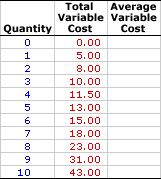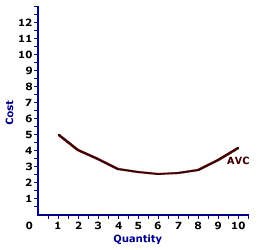Sunday  April 2, 2023
 AmosWEB means Economics with a Touch of Whimsy!KEYNESIAN MODEL: A macroeconomic model based on the principles of Keynesian economics that is used to identify the equilibrium level of, and analyze disruptions to, aggregate production and income. This model identifies equilibrium aggregate production and income as the intersection of the aggregate expenditures line and the 45-degree line. The Keynesian model comes in three basic variations designated by the number of macroeconomic sectors included--two-sector, three-sector, and four sector. The Keynesian model is also commonly presented in the form of injections and leakages in addition to the standard aggregate expenditures format. This model is used to analyze several important topics and issues, including multipliers, business cycles, fiscal policy, and monetary policy.AVERAGE VARIABLE COST:

Total variable cost per unit of output, found by dividing total variable cost by the quantity of output. When compared with price (per unit revenue), average variable cost (AVC) indicates whether or not a profit-maximizing firm should shut down production in the short run. Average variable cost is one of three average cost concepts important to short-run production analysis. The other two are average total cost and average fixed cost. A related concept is marginal cost.
Average variable cost is the total variable cost per unit of output incurred when a firm engages in short-run production. It can be found in two ways. Because average variable cost is total variable cost per unit of output, it can be found by dividing total variable cost by the quantity of output. Alternatively, because total variable cost is the difference between of total cost and total fixed cost, average variable cost can be derived by subtracting average fixed cost from average total cost.

In general, average variable cost decreases with additional production at relatively small quantities of output, then eventually increases with relatively large quantities of output. This pattern is illustrated by a U-shaped average variable cost curve.

Average variable cost, when combined with price, indicates whether or not a firm should shut down production in the short run. If price is greater than average variable cost, then the firm is able to pay all variable cost and a portion of fixed cost. Even though it might be incurring an economic loss, it will lose less by producing that by shutting down production. If, however, price is less than average variable cost, then the firm is better off shutting down production.

### Calculating Average Variable Cost

The standard method of calculating average variable cost is to divide total variable cost by the quantity, illustrated by this equation:
 average variable cost = total variable costquantity of output
An alternative specification for average variable cost is found by subtracting average fixed cost from average total cost:
 average variable cost = average total cost - average fixed cost
An alternative equation computes total variable cost from average variable cost:
 total variable cost = average variable cost x quantity of output

### Stuffed Animal Cost

Average Variable CostInsight into average variable cost can be had using the table to the right, which presents the total variable cost of producing Wacky Willy Stuffed Amigos. The first column is the quantity of Stuffed Amigos rolling off the assembly line, ranging from 0 to 10. The second column is the total variable cost of producing each quantity, ranging from \$0 to \$43. If 5 Stuffed Amigos are produced, then the total variable cost incurred in their production is \$13. The production of 9 Stuffed Amigos, in comparison, incurs a total variable cost of \$31.

Deriving average variable cost is as simple as dividing the second column of total variable cost values by the first column of output quantity values. The average variable cost of producing 1 Stuffed Amigo is relatively easy--divide \$5 by 1 Stuffed Amigo. If the result of this calculation is not readily obvious, click the [One] button.

The average variable cost of two Stuffed Amigos might be a little less obvious, but not much. Click the [Two] button to reveal that the average variable cost is \$4, which is \$8 divided by 2 Stuffed Amigos. A click of the [Three] shows that the average variable cost of producing 3 Stuffed Amigos is \$3.33, \$10 divided by 3. The remaining average variable cost values can be displayed by clicking the [Remaining] button.

With all numbers displayed, what interesting insight into average variable cost can be had?

• First, average variable cost is relatively high for the first Stuffed Amigo produced, then declines. However, it reaches a low, then rises with production of the last few Stuffed Amigos. This is the U-shaped pattern noted above.

• Second, average variable cost remains positive, it never reaches a zero value and never turns negative. The only way for negative average variable cost is for negative total variable cost, which makes no theoretical or practical sense.

### The Average Variable Cost Curve

Average Variable Cost CurveThe relation between average variable cost and the quantity of production can be represented by a curve, such as the one conveniently presented in the exhibit to the right.

The key feature of this average variable cost is the shape. It is U-shaped, meaning it has a negative slope for small quantities of output, reaches a minimum value, then has a positive slope for larger quantities. This U-shape is indirectly attributable to the law of diminishing marginal returns.

The U-shape of the average variable cost curve is indirectly caused by increasing, then decreasing marginal returns (and the law of diminishing marginal returns). The negatively-sloped portion is attributable to increasing marginal returns and the positively-sloped portion is attributable to decreasing marginal returns (and the law of diminishing marginal returns).

 <= AVERAGE TOTAL COST CURVE AVERAGE VARIABLE COST CURVE =>Recommended Citation:

AVERAGE VARIABLE COST, AmosWEB Encyclonomic WEB*pedia, http://www.AmosWEB.com, AmosWEB LLC, 2000-2023. [Accessed: April 2, 2023].

Check Out These Related Terms...

Or For A Little Background...

And For Further Study...
Search Again?PINK FADFLY[What's This?] Today, you are likely to spend a great deal of time searching the newspaper want ads hoping to buy either one of those memory foam pillows or a remote controlled train set. Be on the lookout for celebrities who speak directly to you through your television.Your Complete ScopeThe 1909 Lincoln penny was the first U.S. coin with the likeness of a U.S. President."Before you can inspire with emotion, you must be swamped with it yourself. Before you can move their tears, your own must flow. To convince them, you must yourself believe."-- Sir Winston ChurchillRATSRegression Analysis of Time Series (software)A PEDestrian's Guide Xtra CreditTell us what you think about AmosWEB. Like what you see? Have suggestions for improvements? Let us know. Click the User Feedback link.| | | | | | | | | | |
| | | |

Thanks for visiting AmosWEB